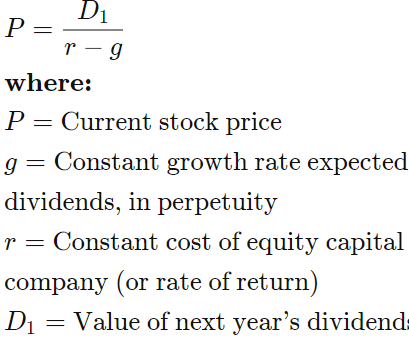# Dismal.Com is a producer of depressing Internet products. The company is currently not paying...

Dismal.Com is a producer of depressing Internet products. The company is currently not paying dividends, but its chief financial officer thinks that starting in 3 years it can pay a dividend of $15 per share, and that this dividend will grow by 20% per year. Assuming that the cost of equity of Dismal.Com is 35%, value a share based on the discounted dividends. ## Dividend Discount Model: This problem entails use of the constant growth dividend discount model (DDM), a widely used method for valuing a stock. The DDM, which is also referred to as the Gordon Growth Model, assumes the fair value of a stock is the sum of all future dividends, discounted to the present day using an appropriate cost of equity. ## Answer and Explanation: The answer is$48.77.

The formula for the DDM is illustrated below.We are given the following information, which will hold three years from now:

D0 = $15.00 g = 20.0% r = 35.0% To solve for P0, we first must determine D1, the dividend expected in the fourth year. It's computed as follows: D1 = D0(1+g) D1 =$15.00(1.20) = $18.00 Now, we can plug the known information into the DDM to solve for P0. The computation is below. P0 =$18.00 / (.35-.20)

P0 = $120.00 However, remember,$120.00 is the value of the stock anticipated in 3 years. To find the present value, we must discount this amount back 3 years at the 35.0% cost of equity. This can be accomplished using the present value formula and computation below.

PV = FV / (1+r)^t

Where,

t = periods

r = discount rate

FV = present value

The computation is as follows:

PV = 120.00 / (1+.35)^3 = \$48.77